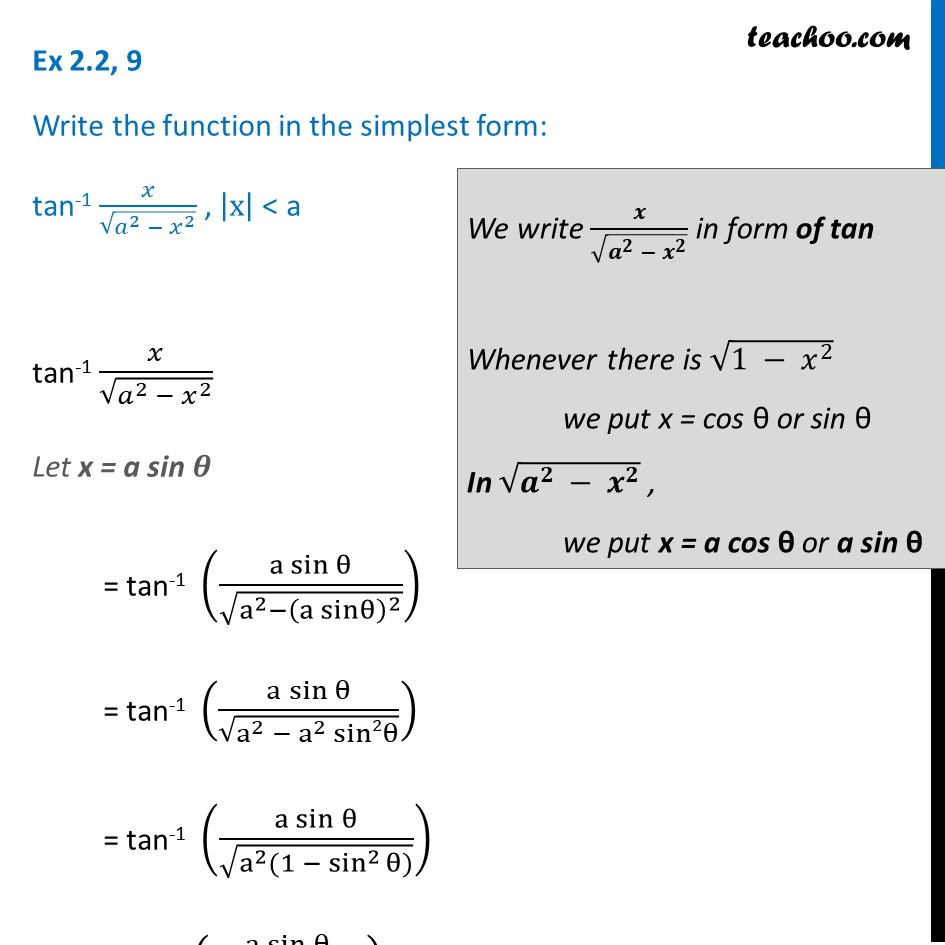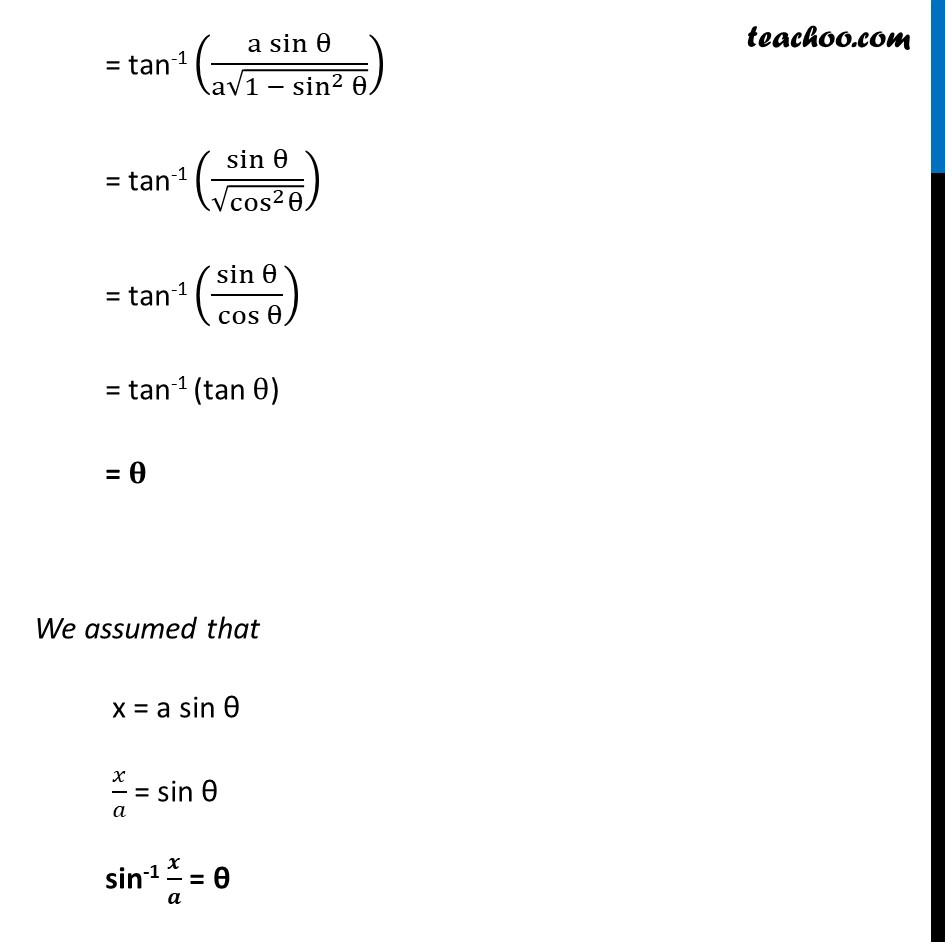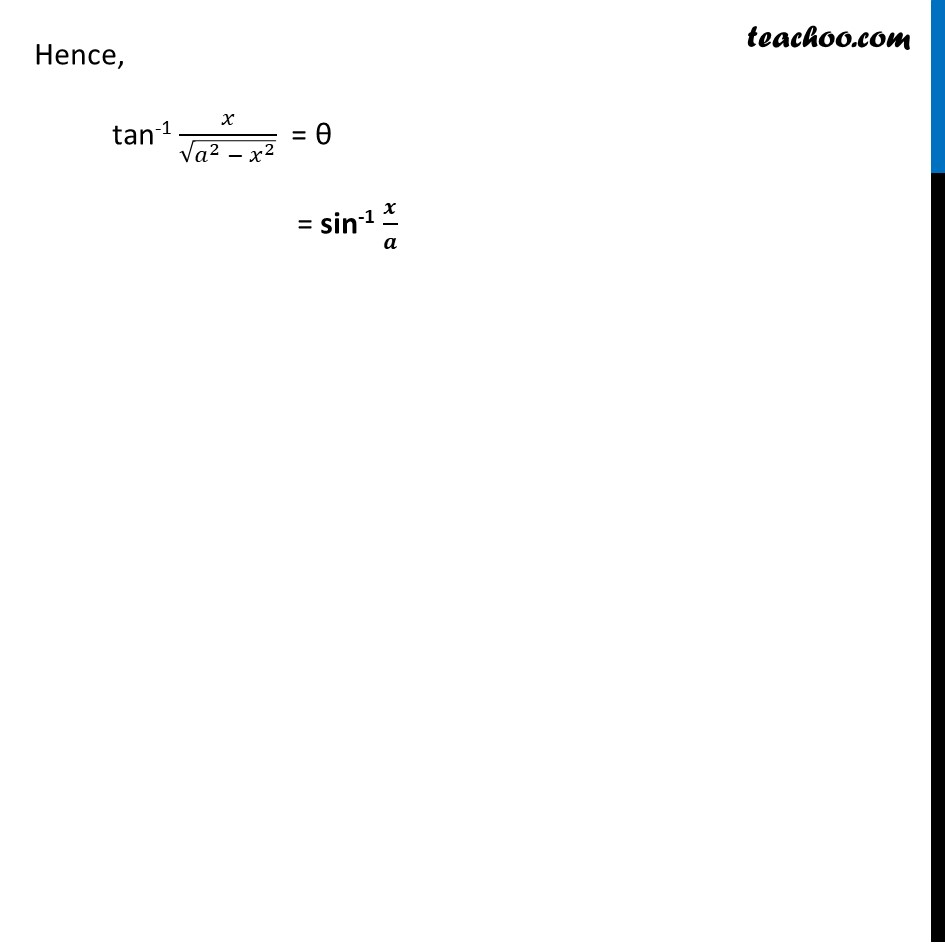Ex 2.2

Chapter 2 Class 12 Inverse Trigonometric Functions
Serial order wiseLearn in your speed, with individual attention - Teachoo Maths 1-on-1 Class

### Transcript

Ex 2.2, 6 Write the function in the simplest form: tan-1 𝑥/√(𝑎^2 − 𝑥^2 ) , |x| < a tan-1 𝑥/√(𝑎^2 − 𝑥^2 ) Let x = a sin 𝜽 = tan-1 ((a sin⁡θ)/√(a^2−(a sinθ)^2 )) = tan-1 ((a sin⁡θ)/√(a^2 − a^2 sin2θ)) = tan-1 ((a sin⁡θ)/√(a^2 (1 − sin^2 θ))) = tan-1 ((a sin⁡θ)/(a√(1 − sin^2 θ))) = tan-1 (sin⁡θ/√(cos^2 θ)) We write 𝒙/√(𝒂^𝟐 − 𝒙^𝟐 ) in form of tan Whenever there is √(1 − 𝑥^2 ) we put x = cos θ or sin θ In √(𝒂^𝟐 − 𝒙^𝟐 ) , we put x = a cos θ or a sin θ = tan-1 ((a sin⁡θ)/(a√(1 − sin^2 θ))) = tan-1 (sin⁡θ/√(cos^2 θ)) = tan-1 (sin⁡θ/〖 cos〗⁡θ ) = tan-1 (tan θ) = 𝛉 We assumed that x = a sin θ 𝑥/𝑎 = sin θ sin-1 𝒙/𝒂 = θ Hence, tan-1 𝑥/√(𝑎^2 − 𝑥^2 ) = θ = sin-1 𝒙/𝒂